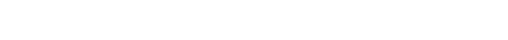# 1.2.3 Thermal Properties of GaN

1.2.3Thermal Properties of GaNIn a similar vein,GaN and other allied group III nitride semiconductors are grown
at high temperatures and also subjected to increased junction temperatures during
operation of devices such as amplifiers and light emitting devices. As such, the
structures are subjected to thermal variations as well. In this context, it is imperative
to have knowledge of the thermal expansion coefficients, which are termed as TEC.
Assuming that these figure remain the same with temperature, the linear expansion
coefficients for the a and c parameters are tabulated in Tables 1.10 and 1.28 for
heteroepitaxial GaN. However, it is instructive to know the temperature dependence
of these parameters, which is shown in Figure 1.16.
Being grown on various substrates with different thermal expansion coefficients
leads to different dependencies of the lattice parameter on temperature. Temperature
dependence of GaN lattice parameter has been measured for a bulk crystal (grown at
high pressure) with a high free-electron concentration (5 · 1019 cm3), a slightly
strained homoepitaxial layer with a low free-electron concentration (about 1017 cm3),
and a heteroepitaxial layer (also with a small electron concentration) on sapphire .
The results of such study are tabulated in Table 1.28.
It can be seen that the bulk sample with a high free-electron concentration exhibits
a thermal expansion that is about 3% higher as compared to the homoepitaxial layer.Figure 1.16 Wz GaN coefficient of linear thermal expansion
versus temperature for basal plane (a||), a parameter, and out of
the basal plane (a?), c parameter, directions .

As for the case of the heteroepitaxial layer on sapphire, the thermal expansion of the
substrate affects the dependence of the lattice parameter on temperature.
Various spectroscopic techniques, such as Auger electron spectroscopy, X-ray
photoemission spectroscopy (XPS), and electron energy loss spectroscopy (EELS)
have been very useful for the study of surface chemistry of GaN. Building on earlier
investigations of the thermal stability of GaN by Johnson et al.  and employing

Table 1.28 Lattice parameters for GaN samples at various
temperatures (lattice parameters c were measured with accuracy
of 0.0002 Å, lattice parameter a with accuracy of 0.0005 Å) .the aforementioned techniques, the thermal stability and dissociation of GaN have
been examined further. As indicated earlier, the materials characteristics depend, to a
large extent, on defects and impurities, which in turn depend somewhat on growth
conditions. Because of this the materials, obtained from various sources, studied in
various laboratories exhibit different characteristics. This led to inconsistent results
from different laboratories. While some experimental studies on GaN stability
conducted at high temperatures suggested that significant weight losses occur at
temperatures as low as 750 C, others contradicted this proposition and suggested
that no significant weight loss should occur even at a temperature of 1000 C. Sime
andMargrave  followed the investigation by Johnson et al.  by studying the
evaporation of GaN and Ga metal in the temperature range 900–1150 C under
atmospheric pressure in N2, NH3, and H2 environments with an emphasis on the
formation and decomposition equilibrium.
The heat of evaporation was determined and the existence of (GaN)x polymers in the
gas phase was suggested. Morimoto  and Furtado and Jacob  observed that
GaN is less stable in an HCl or H2 atmosphere than in N2. Some controversy exists
regarding the process steps that dictate the decomposition of GaN. Using mass
spectroscopy, Gordienko et al.  noted that (GaN)2 dimers are the primary
components of decomposition. Others [187,188] found only N2 þ and Gaþ to be the
primary components in the vapor over GaN. On the basis of measurements of the
apparent vapor pressure, Munir and Searcy  calculated the heat of sublimation of
GaN to be 72.40.5 kcalmol1. Thurmond and Logan  determined the equilibrium
N2 pressure of GaN as a function of temperature by measuring the partial
pressure ratios existing in a (H2CNH3) gas mixture streaming over Ga and GaN.
Thermal stability of GaN was taken up later by Karpinski et al.  with a detailed
investigation of the problemat high temperatures and under pressure up to 60 kbar by
employing a tungsten carbide anvil cell activated by a gas pressure technique.
The bond strength in gallium nitride is high with bonding energy of
9.12 eV/molecule , particularly as compared to the more conventional semiconductors
such asGaAs, which has a bonding energy of 6.5 eV/atom pair.As a result,
the free energy of GaN is very low in relation to the reference states of the free N and
Ga atoms. However, the N2 molecule is also strongly bonded with 4.9 eV/atom.
Therefore, the free energies of the constituents of GaN (Ga and N2) at their normal
states are close to that of the GaN crystal as illustrated in Figure 1.17, where the free
energy ofGaN(1 mol) and the free energy of the sum of its constituents (Gaþ1/2N2)
are shown as a function of temperature and N2 pressure. As the temperature
increases, the Gibbs free energy, G(T), of the constituents decreases faster than G
(T) of the GaN crystal. More importantly, GaN becomes thermodynamically unstable
at high temperatures. The crossing of G(T) curves determines the equilibrium
temperature where GaN coexists with its constituents at a given N2 pressure. The
application of pressure increases the free energy of the constituents more than G(T)
of the GaN crystal, which causes the equilibrium point to shift to higher temperatures,
increasing the range of GaN stability.
The data on phase diagrams ofGaNare limited and contradictory by reason of high
melting temperatures (Tm) and high nitrogen dissociation pressures (Pdis
N2 ). DissociationFigure 1.17 Gibbs free energy of GaN and its constituents as a
function of temperature and pressure .process of dissociation. Expanded equilibrium vapor pressure data inclusive of GaAs
and InP in addition to the three nitride binaries reported byMatsuoka  are shown
in Figure 1.19. Melting points and other thermodynamic characteristics of III-N
compounds are given in Tables 1.10, 1.16, and 1.20 as compiled by Popovici and
Morkoc  as well as those collected from various sources as indicated in the
pertinent tables.
Investigations utilizing epitaxial thin films of GaN, as well as AlN and InN, have
been conducted by Ambacher et al. , who heated the samples in vacuum and
recorded the partial pressure of relevant gases with a quadrupole mass spectrometer.
Desorption spectra were then analyzed  to find the binding energies of various
desorbed species as well as the thermal stability of the sample for a given thermal
treatment. As expected, the nitrogen partial pressure increases exponentially above
TE¼850 C for GaN underscoring the point that the decomposition temperature in
vacuum is much lower than the melting point shown in Figure 1.18. The rate ofFigure 1.19 Equilibrium vapor pressure of N2 over AlN, GaN
and InN, the sum of As2 and As4 over GaAs, and the sum of P2
and P4 over InP .

nitrogen evolutionF(N) was set equal to the rate of decomposition, and the slope of ln
[F(N)] versus 1/T gives the effective activation energy of the decomposition in
vacuum as compared to those shown in Figure 1.18. The decomposition rate equals
the desorption of one monolayer every second (FN¼1.5 · 1015 cm2 s1) at 970 C,
and the activation energy of the thermally induced decomposition is determined to be
EMN¼3.9 eV (379 kJ mol1) for GaN.
Despite some disagreement, as mentioned above, investigations of the equilibrium
nitrogen overpressure versus temperature, PN2–T, for GaN [92,190,201], including a
very complete and consistent set of data obtained by Karpinski et al. [191,202] have set
the stage for bulk template growth as well as setting benchmarks for growth ofGaN by
nonequilibrium methods. Those authors employed direct synthesis and decomposition
experiments and used the gas pressure technique (for pressures up to 20 kbar)
and the high-pressure anvil method beyond the reach of gas pressure technique
(up to 70 kbar). The results of these experiments are shown in Figure 1.20 [203,204].
The message in the form of N2 partial pressure is that one must stay below the
decomposition curve. This means that the selection of GaN synthesis temperature
directly depends on the pressure that the vessel can provide. For example, if a pressure
of 20 kbar is all that is available, then the temperature should be kept below about
1660  C. For a review of the stability of GaN as well as the growth GaN templates, the
reader is referred to Ref. .
As alluded to earlier, nitride semiconductors in general and GaN in particular are
considered for high-power/high-temperature electronic and optoelectronic devicesFigure 1.20 N2 partial pressure as a function of temperature GaN.
Ref. , originally in Refs [203,204].

where thermal dissipation is a key issue. Device applications assure that the thermal
conductivity (k) is an extremely important material property. Thermal conductivity is a
kinetic property determined by contributions from the vibrational, rotational, and
electronic degrees of freedom, and as such it is related to the mechanical properties of
the material. However, for convenience, this property is generally categorized under
the thermal properties of nitrides in this book. The electronic thermal conductivity
contribution is negligible for carrier concentrations1019cm3.The heat transport is
predominantly determined by phonon–phonon Umklapp scattering, and phonon
scattering by point and extended defects such as vacancies (inclusive of the lattice
distortions caused by them), impurities,and isotopefluctuations (massfluctuation) as
elaborated on by Slack et al. . For pure crystals, phonon–phonon scattering, which
is ideally proportional to T1 above the Debye temperature, is the limiting process.
The lattice contribution (phonon scattering) to the thermal conductivity, k, in a
pure solid is obtained from the kinetic theory as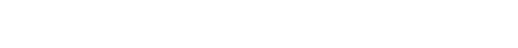where T is the temperature, vs is the velocity of sound (nearly independent of
temperature), Clattice(T) is the lattice specific heat, and L(T) is the phonon mean free
length. In nearly all materials, the thermal conductivity, k(T), first increases with
temperature, reaches a maximum (kmax) at some characteristic temperature Tch, and

then decreases. At low temperatures, L is relatively long and is dominated by extrinsic
effects such as defects and/or finite crystal size and Clattice(T)(T/yD)3, where yD is
the Debye temperature. As the temperature increases, Clattice(T) begins to saturate
and the intrinsic temperature-dependent Umklapp processes become dominant,
leading to a decrease in L.
The other contribution, the electronic contribution, to the thermal conductivity is
negligible for carrier concentrations 1019 cm3. It can be expressed as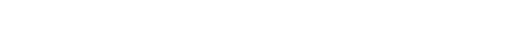where n is the carrier density, kB is the Boltzmann constant, telectr is the scattering
time of the electrons, and mc is the conduction band effective mass.The heat current Q creates a temperature gradient of DT across the wafer. The k
value is calculated using k¼(P/DT)(L/A), where P is the power (¼voltage · current)
supplied to the heater and A represents the cross-sectional area of the sample.
Although the technique sounds simple, its accuracy depends very critically on
making sure that the heat conduction is through the specimen and along the
direction in which the temperature gradient is measured. To make certain that heat
is transferred in the said direction only, the radiation losses must be minimized as
well as making sure that the electrical wires used do not remove heat. To this end, the
sample is placed in a turbo pumped vacuum to eliminate conduction and convection
through the surrounding medium.Heat losses via conduction through the wires are
minimized using long (10 cm), thin (<100 mm) wires of low thermal conductivity,
typically chromel/constantan thermocouple and heater wires. Radiation losses areFigure 1.21 Schematic representation of the four-probe
thermoelectric measurement setup used to measure the thermal
conductivity of freestanding GaN .

minimized by surrounding the sample with a heat shield anchored thermally to the
cold tip of the cryostat. By carefully designing this shield, Slack et al.  were able to
reduce the total heat loss to the order of 1–2mWK1 at room temperature. Because
the radiation losses follow T3 dependence, they die off rather quickly below room
temperature. For a sample with a thermal conductivity of 1Wcm1 K1, cross section
of 1 ·3mm2, and thermocouple probe separation of 5 mm, the thermal conductance
is about 60mWK1, so the heat losses are less than 5%. Fortunately, for wide
bandgap semiconductors such asGaN the thermal conductivities are high enough so
that the heat conduction is mainly through the sample, reducing the measurement
error. Just as a reference point, for samples of lower thermal conductance (either
lower conductivity or thinner), the heat losses can become important near room
temperature. Thus, the samples for lower thermal conductivity materials (e.g.,
glasses or thermoelectric alloys) usually need to be short with large cross-sectional
GaN is shown in Figure 1.22. From that temperature dependence and assuming
the heat dissipation is through acoustic phonons, a Debye temperature of yD
550 K
was deduced, which compares with 650 K reported by Slack et al. .
As can be seen in Figure 1.22, the measured thermal conductivity of GaN in the
temperature range of 80–300K has a temperature power dependence of 1.22. This
slope is typical of pure adamantine crystals below the Debye temperature indicating
acoustic phonon transport where the phonon–phonon scattering is a combination ofFigure 1.22 The thermal conductivity of 200 mmthick freestanding
GaN sample (Samsung) as a function of temperature. The dashed
line indicates calculation using the boundary scattering limit for a
phonon mean free path of 500 mm. Also shown is the
T1.22dependence between about 80 and 300 K, and earlier results
of Sichel and Pankove  measured using a 400 mm HVPE
sample. Courtesy of Slack and Morelli .

acoustic–acoustic and acoustic–optic interactions. This temperature dependence
strongly suggests that the thermal conductivity depends mainly on intrinsic phonon–
phonon scattering and not on phonon–impurity scattering. Keep in mind that
the net electron concentration in the measured film is about 1016 cm3 and the hole
concentration is in the 1015 cm3 range. In addition, the dislocation density is low,
about 106 cm2. It should be pointed out that the thermal conductivity degrades with
increased dislocation density, particularly above 107 cm2, with a slope of 0.4W
cm1 K1 per decade dropping down to slightly above 1Wcm1 K1 for a dislocation
density of mid-109cm2. The dashed curve at low temperatures in Figure 1.22 has
been calculated for boundary scattering assuming a mean free path of 500 mm .
The mean free path is comparable with the average sample diameter, which indicates
that impurity scattering in this region is not dominant either.
The thermal conductivity of GaN is a tensor quantity and has two principal values
k? and k|| perpendicular and parallel to the c-axis, respectively, that is, in-plane and
out-of-plane values. The anisotropy in the sound velocity, which relates to the phonon
propagation velocity, has been calculated by Polian et al.  in the form of n?¼5.56
· 105 cm s1 and n||¼5.51 · 105 cm s1 for in-plane and out-of-plane directions,
respectively. These values are smaller than the measured values, reported by
Deger et al. , of n?¼ 8.02 · 105 cms1 and n||¼7.79 · 105 cms1 for in-plane

and out-of-plane directions, respectively. The in-plane and out-of-plane sound
velocities reported in Ref  are tabulated in Tables 1.7 and 1.9 for wurtzitic and
zinc blende phases of GaN. Because the difference is negligible and the anharmonicity
producing the phonon–phonon scattering is not discernible, one can conclude
that in-plane thermal conductivity measurements are a good representative of k in
GaN .
A newer method, named the scanning thermal microscopy (SThM) , has been
developed to measure thermal conductivity and is purported to provide nondestructive,
absolute measurements with a high spatial/depth resolution of about 2–3 mm.
Thermalimagingis achievedusing a resistivethermal elementincorporated at theend
of a cantilever/AFM-type feedback asshowninFigure 1.23.Theresistive tip formsone
element of a Wheatstone bridge as shown in Figure 1.24. The spatial/depth resolution
is estimated to be 2–3 mm for GaN and AlN. Upon contact with the sample, the tip
tends to cooldown due to heat conduction into the sample, which is related to its
thermal conductivity, k. The bridge circuit applies a compensating voltage (Uout) to
maintain its target operating temperature. The feedback signal for constant resistance
is a measure of the thermal conductivity of the material with which the tip is in contact,
specificallyV2
out is proportional tok because powerdissipation is themechanismhere.
Measurements of the absolute values of k are based on a calibration procedure. This
simply comprises calibrating the feedback signal,V2
out, for a constant thermal element
resistance against that for samples with known conductivities such as GaSb, GaAs,
InP, Si, and Al metal, as shown in Figure 1.25. The influence of the surface roughness
on the effective thermal conductivity is of concern. For a perfectly flat surface,Figure 1.23 An artists view of the scanning thermal microscope.
Patterned after D.I. Florescu and F.H. Pollak. (Please find a color
version of this figure on the color tables.)Figure 1.24 (a) Wheatstone bridge arrangement
in which the tip temperature is kept constant
before and after contact with the material whose
thermal conductivity is being measured. The
feedback signal Uout is related to thermal
conductivity, k. A calibration against known
samples such as Si, GaAs, GaP, and so on, leads
to absolute values of k. (b) Schematic diagram of
heat dissipation into the sample from the tip.
Courtesy of D.I. Florescu and F.H. Pollak.

the contact between the probe tip (radius of curvature 1 fm) and the sample surface
is very small. For rough surfaces, however, the tip could impinge on a valley- or
hillocklike feature with the valley/hillock leading to increased/decreased thermal
signal accompanied by a corresponding change in the measured effective thermal
conductivity.Figure 1.25 The feedback signal, V2
out, which is a measure of the
thermal conductivity of the material under test, for a constant
thermal element resistance for samples with known conductivities
such as GaSb, GaAs, InP, Si, and Al metal. Courtesy of D.I.
Florescu and F.H. Pollak.

The SThM method has been applied to the measurement of the room-temperature
thermal conductivity on both fully and partially coalesced epitaxial lateral overgrown
GaN/sapphire (0 0 0 1) samples . As expected, a correlation between low threading
dislocation density and high thermal conductivity values was established. The
reduction in the thermal conductivity with increased dislocation density is expected
scattering in the material. In fact, due to the high defect concentrations in early
films, the thermal conductivity value measured was 1.3Wcm1 K1 . Using this
method, the highest GaN k values, 2.0–2.1Wcm1K1, were found in the regions of
the samples that were laterally grown and thus contained the lowest density of
threading dislocations. This compares with a value of 2.3Wcm1 K1 in a freestanding
sample measured by the steady-state four-probe method discussed earlier. Even
then, it falls short of the predictions by Witek .
An explanation for the dramatic increase from to k1.3Wcm1 K1 for the
early samples to 2.3Wcm1 K1for the freestanding sample, as iterated above is
most likely related to extended defect concentration (Dd) and the differences in
background doping. The effect of dislocation density on the thermal conductivity
has been calculated by Kotchetkov et al. . The dislocation density in the thick
film measured by Sichel and Pankove was between 109 and 1010 cm2, while the
freestanding sample exhibited densities of less than 106 cm2 near the top surface
(Ga-polarity) and 107 cm2 near the bottom surface (N-polarity). Kotchetkov et al.
showed that k remains fairly independent of Dd up to some characteristic
value Dchar
d after which it decreases about a factor of 2 for every decade of
increase in Dd.
The thermal conductivity has also been correlated to doping levels in HVPE
n-GaN/sapphire (0 0 0 1) by SThM on two sets of samples [211,212]. In both sets of
data, the thermal conductivity decreased linearly with log n, n being the electron
concentration, the variation being about a factor of 2 decrease in k for every decade
increase in n. Significantly, it was concluded that the decrease in the lattice
contribution to k, due to increased phonon scattering from impurities and free
electrons, predominates the increase in the electronic contribution. Also, a correlation
between the film thickness and the improved thermal conductivity was found,
which is consistent with the observed general reduction of both extended (dislocations)
and point defects with film thickness .
The k values at 300 K before and after plasma-induced effects on a series of n-GaN/
sapphire (0 0 0 1) samples fabricated by HVPE were also measured . The sample
thicknesses were 505 mm and the carrier concentrations were 8 · 1016 cm3, as
determined byHall effect measurements. The thermal conductivity before treatment
was found to be in the 1.70–1.75Wcm1 K1 range, similar to that previously
reported for HVPE material with this carrier concentration and thickness [211,212].
The k value was reduced, however, when the samples were processed under constant
Ar gas flow and pressure for a fixed period of time (5 min). The only variable
processing parameter was the DC bias voltage (125–500 V). After the initial 125V
procedure, k exhibited a linear decrease with theDCvoltage in the investigated range.
At 125 V, the thermal conductivity was only slightly less (k1.65Wcm1 K1)

than the untreated case. The values of k had dropped to 0.3Wcm1 K1 for the
500 V case.
To a first extent, the temperature dependence of the specific heat of WzGaN(Cp) at
constant pressure can be expressed by phenomenological expression . In this
vein, the specific heat Cp of Wz GaN at constant pressure for 298 K<T<1773 K can
be expressed as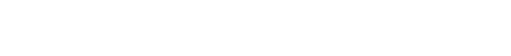However, this expression is very simplistic, as will be seen below. As already
mentioned, free electrons (very effective at low temperatures), impurities, defects
(inclusive of point defects), and lattice vibrations contribute to specific heat. If GaN
with negligible free-electron concentration and defects were available, only the lattice
contribution would be considered, which is also the case in texts . The specific
heat ofWzGaNhas been studied by Koshchenko et al.  in the temperature range
of 5–60 K and also by Demidienko et al.  in the temperature range of 55–300 K
and discussed by Krukowski et al. [88,127]. The Debye expression for the temperature
dependence of specific heat in a solid at a constant pressure (Cp) can be expressed
aswhere xD qD=T and R=8.3144 Jmol1K1 is the molar gas constant. The coefficient
in front of the term R has been multiplied by 2 to take into account the two
constituents making up the binary GaN. By fitting the measured temperature-dependent
heat capacity to the Debye expression, one can obtain the Debye temperature yD
specific to heat capacity. The experimental data of Demidienko et al. and Krukowski et
al. are plotted in Figure 1.26. Also shown in the figure is the calculated specific heat
using the Debye expression for Debye temperatures of 500, 600, 700, and 800 K. It is
clear that the quality of the data and/or sample prevents attainment of a good fit
between the experimental data and Equation 1.16. Consequently, a Debye temperature
with sufficient accuracy cannot be determined. It is easier to extract a Debye temperature
using data either near very lowtemperatures or well belowthe Debye temperature
where the specific heat has a simple cubic dependence of temperature :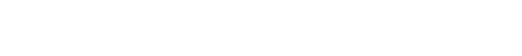Unfortunately, the GaN samples contain large densities of free carriers and defects
that compromise the application of the Debye specific heat expression. Consequently,
a good fit to the data is not obtained and the Debye temperature so extracted is not as
dependable as desired.
There is a spread in the reported Debye temperatures for GaN, yD, in the range of
about 600 to 700 K. Slack  estimated a value of 600 K at 0 K by utilizing the more
established Debye temperatures forBeO and AlN. This compares with 550 KdeducedFigure 1.26 Molar specific heat at constant
pressure, Cp (cal mol1 K1), of GaN versus
temperature. Open circles represent the
experimental data. The solid lines are calculation
based on the Debye model for Debye
temperatures, yD, of 500, 600, 700, and 800 K.
Unfortunately, it is difficult to discern a Debye
temperature that is effective over a wide
temperature range because a large concentration
of defects and impurities is present in GaN.
However, a value of 600 K estimated by Slack is
used commonly. The data are taken from
Refs [215,216], as compiled in Ref. . (Please
find a color version of this figure on the color
tables.)

from heat transfer due to acoustic phonons, as mentioned above. Because the samples
used in these measurements contained defects and large density of free electrons, the
dispersion among the data and Debye expression is attributed to defects at high
temperaturesandfree electrons atlowtemperatures.Elastic properties ofGaNcanalso
be used to deduce the Debye temperature. In this vein, Raman scattering measurements
yielded a Debye temperature of yD=650 K.Calculations since the estimate
of Slack  yielded a range of 620–690 K .
Thermodynamic properties of Wz GaN have been reported by Elwell and
Elwell . From the reaction